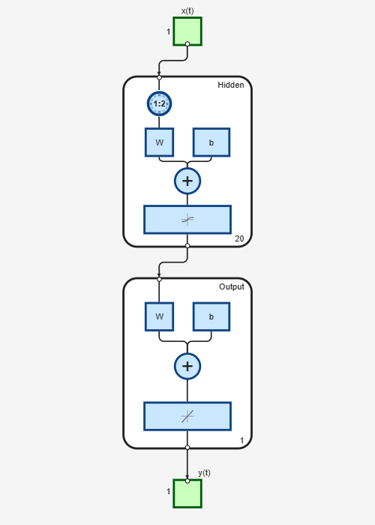# preparets

Prepare input and target time series data for network simulation or training

## Syntax

``[Xs,Xi,Ai,Ts,EWs,shift] = preparets(net,Xnf,Tnf,Tf,EW)``

## Description

example

````[Xs,Xi,Ai,Ts,EWs,shift] = preparets(net,Xnf,Tnf,Tf,EW)` takes these arguments: `net` — Neural network`Xnf` — Non-feedback inputs`Tnf` — Non-feedback targets`Tf` — Feedback targets`EW` — Error weights (optional) and returns these arguments: `Xs` — Shifted inputs`Xi` — Initial input delay states`Ai` — Initial layer delay states`Ts` — Shifted targets`EWs` — Shifted error weights`shift` — The number of timesteps truncated from the front of `X` and `T` in order to properly fill `Xi` and `Ai`. This function simplifies the normally complex and error prone task of reformatting input and target time series. It automatically shifts input and target time series as many steps as are needed to fill the initial input and layer delay states. If the network has open-loop feedback, then it copies feedback targets into the inputs as needed to define the open-loop inputs.Each time a new network is designed, with different numbers of delays or feedback settings, `preparets` can reformat input and target data accordingly. Also, each time a network is transformed with `openloop`, `closeloop`, `removedelay` or `adddelay`, this function can reformat the data accordingly.```

## Examples

collapse all

This example shows how to prepare data for open-loop and closed-loop networks.

Create a time-delay network with 20 hidden neurons, then train and simulate it.

```[X,T] = simpleseries_dataset; net = timedelaynet(1:2,20); [Xs,Xi,Ai,Ts] = preparets(net,X,T); net = train(net,Xs,Ts); view(net)````Y = net(Xs,Xi,Ai);`

Design a NARX network. The NARX network has a standard input and an open-loop feedback output to an associated feedback input.

```[X,T] = simplenarx_dataset; net = narxnet(1:2,1:2,20); [Xs,Xi,Ai,Ts] = preparets(net,X,{},T); net = train(net,Xs,Ts,Xi,Ai);````view(net)``y = net(Xs,Xi,Ai);`

Now convert the network to closed loop, and reformat the data to simulate the network’s closed-loop response.

```net = closeloop(net); view(net)``````[Xs,Xi,Ai] = preparets(net,X,{},T); y = net(Xs,Xi,Ai);```

## Input Arguments

collapse all

Input network, specified as a network object. To create a network object, use for example, `feedforwardnet` or `narxnet`.

Non-feedback input data (inputs not associated with an open loop feedback output), specified as cell array.

Target data for non-feedback outputs, specified as a cell array.

Target data for outputs with feedback, specified as a cell array.

Error weights, specified as a cell array.

## Output Arguments

collapse all

Shifted inputs, returned as a cell array.

Initial input delay states, returned as cell array.

Initial layer delay states, returned as a cell array.

Shifted targets, returned as a cell array.

Shifted error weights, returned as a cell array.

Number of timesteps truncated from the front of `X` and `T` in order to properly fill `Xi` and `Ai`, returned as a scalar.

## Version History

Introduced in R2010b